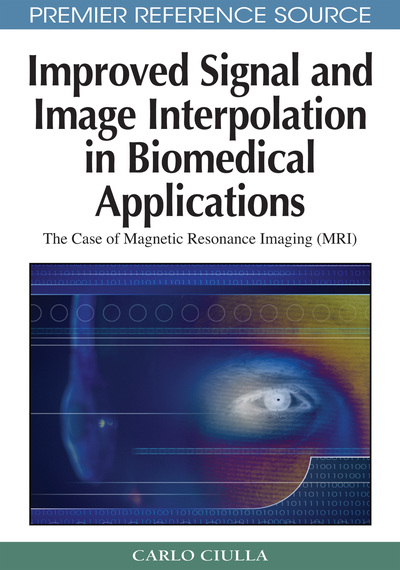# The Conception of the Sub-Pixel Efficacy Region

Carlo Ciulla (Lane College, USA)
DOI: 10.4018/978-1-60566-202-2.ch004
Available
\$37.50
No Current Special Offers

## Abstract

This chapter presents the mathematical deduction of the Sub-pixel Efficacy Region (SRE) for the case of the trivariate liner interpolation function. The questions that the reader may have at this point are: (i) what is the need of defining the Sub-pixel efficacy Region once again? And (ii) why the Sub-pixel Efficacy Region is to be calculated on the basis of the Intensity-Curvature Functional?
Chapter Preview
Top

## Sub-Pixel Efficacy Region

The Sub-Pixel Efficacy Region is derived through the study of the Intensity-Curvature Functional, particularly through the process of finding the extremes of ΔE. Based on the results of Chapter III, the Intensity-Curvature Functional is herein calculated:

ΔE(x, y, z) = Eo / EIN(1) EIN = EIN(x, y, z) = EINxy) + EINzx) + EINyz) = x y z x y z ∫ ∫ ∫ h(x, y, z) (¶2 (h(x,y,z)) /¶x¶y) dx dy dz + ∫ ∫ ∫ h (x, y, z) (¶2(h(x,y,z)) /¶z¶x) dx dy dz + 0 0 0 0 0 0x y z ∫ ∫ ∫ h (x, y, z) (¶2(h(x,y,z)) /¶y¶z) dx dy dz (2)0 0 0 Eo = Eo(x, y, z) = Eoxy) + Eozx) + Eoyz) = x y z ∫ ∫ ∫ f (0, 0, 0) (¶2 (h(x,y,z))/¶x¶y + ¶2 (h(x,y,z))/¶y¶z + ¶2 (h(x,y,z)) /¶x¶z) (0, 0, 0) dx dy dz (3)0 0 0

It is due to clarify that above integrals are calculated from (0, 0, 0) (the origin of the coordinate system of the voxel, assumed at the location of the node of intensity f(0, 0, 0)) to anywhere (x, y, z) in the voxel.

For the rest of the interpolation functions treated in the book, the integrals of the intensity-curvature terms are calculated from the origin of the coordinate system in between two nodes (1D) or the pixel (2D) respectively, to anywhere within the intra-nodal distance or the pixel.

The calculation shown in equations (2) and (3) is different from the one previously seen in Chapter III, where the integrals where calculated from (-x/2, -y/2, -z/2) to (x/2, y/2, z/2) such to quantify the energy level of the signal (image) obtained before and after interpolation. In this chapter the concept of energy is temporarily subsidized by the concept of Intensity-Curvature content embedded into the model interpolation function at a given re-sampling location (x, y, z) in the voxel.

## Complete Chapter List

Search this Book:
Reset